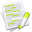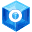#wx.GraphicsPath¶

A wx.GraphicsPath is a native representation of a geometric path.

The contents are specific and private to the respective renderer. Instances are reference counted and can therefore be assigned as usual. The only way to get a valid instance is by using wx.GraphicsContext.CreatePath or wx.GraphicsRenderer.CreatePath .

##Class Hierarchy¶Inheritance diagram for class GraphicsPath:

##Methods Summary¶

 AddArc Adds an arc of a circle. AddArcToPoint Adds an arc (of a circle with radius r) that is tangent to the line connecting current point and (x1, y1) and to the line connecting (x1, y1) and (x2, y2). AddCircle Appends a circle around (x,y) with radius r as a new closed subpath. AddCurveToPoint Adds a cubic bezier curve from the current point, using two control points and an end point. AddEllipse Appends an ellipse fitting into the passed in rectangle as a new closed subpath. AddLineToPoint Adds a straight line from the current point to (x,y). AddPath Adds another path onto the current path. AddQuadCurveToPoint Adds a quadratic bezier curve from the current point, using a control point and an end point. AddRectangle Appends a rectangle as a new closed subpath. AddRoundedRectangle Appends a rounded rectangle as a new closed subpath. CloseSubpath Closes the current sub-path. Contains GetBox Gets the bounding box enclosing all points (possibly including control points). GetCurrentPoint Gets the last point of the current path, (0,0) if not yet set. GetNativePath Returns the native path (CGPathRef for Core Graphics, Path pointer for GDIPlus and a cairo_path_t pointer for cairo). MoveToPoint Begins a new subpath at (x,y). Transform Transforms each point of this path by the matrix. UnGetNativePath Gives back the native path returned by GetNativePath because there might be some deallocations necessary (e.g.

##Class API¶

class wx.GraphicsPath(GraphicsObject)

A GraphicsPath is a native representation of a geometric path.

### Methods¶

AddArc(self, *args, **kw)

Adds an arc of a circle.

The circle is defined by the coordinates of its centre (x, y) or c and its radius r. The arc goes from the starting angle startAngle to endAngle either clockwise or counter-clockwise depending on the value of clockwise argument.

The angles are measured in radians but, contrary to the usual mathematical convention, are always clockwise from the horizontal axis.

If for clockwise arc endAngle is less than startAngle it will be progressively increased by 2pi until it is greater than startAngle. If for counter-clockwise arc endAngle is greater than startAngle it will be progressively decreased by 2pi until it is less than startAngle.

If there is a current point set, an initial line segment will be added to the path to connect the current point to the beginning of the arc.Overloaded Implementations:

AddArc (self, x, y, r, startAngle, endAngle, clockwise)

Parameters
• x (wx.Double) –

• y (wx.Double) –

• r (wx.Double) –

• startAngle (wx.Double) –

• endAngle (wx.Double) –

• clockwise (bool) –

AddArc (self, c, r, startAngle, endAngle, clockwise)

Parameters
• c (wx.Point2D) –

• r (wx.Double) –

• startAngle (wx.Double) –

• endAngle (wx.Double) –

• clockwise (bool) –

AddArcToPoint(self, x1, y1, x2, y2, r)

Adds an arc (of a circle with radius r) that is tangent to the line connecting current point and (x1, y1) and to the line connecting (x1, y1) and (x2, y2).

If the current point and the starting point of the arc are different, a straight line connecting these points is also appended. If there is no current point before the call to AddArcToPoint this function will behave as if preceded by a call to MoveToPoint(0, 0). After this call the current point will be at the ending point of the arc.Parameters
• x1 (wx.Double) –

• y1 (wx.Double) –

• x2 (wx.Double) –

• y2 (wx.Double) –

• r (wx.Double) –

AddCircle(self, x, y, r)

Appends a circle around (x,y) with radius r as a new closed subpath.

After this call the current point will be at ( x+ r , y).

Parameters
• x (wx.Double) –

• y (wx.Double) –

• r (wx.Double) –

AddCurveToPoint(self, *args, **kw)Overloaded Implementations:

AddCurveToPoint (self, cx1, cy1, cx2, cy2, x, y)

Adds a cubic bezier curve from the current point, using two control points and an end point.

If there is no current point before the call to AddCurveToPoint this function will behave as if preceded by a call to MoveToPoint(cx1, cy1).

Parameters
• cx1 (wx.Double) –

• cy1 (wx.Double) –

• cx2 (wx.Double) –

• cy2 (wx.Double) –

• x (wx.Double) –

• y (wx.Double) –

Adds a cubic bezier curve from the current point, using two control points and an end point.

If there is no current point before the call to AddCurveToPoint this function will behave as if preceded by a call to MoveToPoint(c1).

Parameters

AddEllipse(self, x, y, w, h)

Appends an ellipse fitting into the passed in rectangle as a new closed subpath.

After this call the current point will be at ( x+ w , y+ h/2 ) .

Parameters
• x (wx.Double) –

• y (wx.Double) –

• w (wx.Double) –

• h (wx.Double) –

AddLineToPoint(self, *args, **kw)Overloaded Implementations:

Adds a straight line from the current point to (x,y).

If current point is not yet set before the call to AddLineToPoint this function will behave as MoveToPoint .

Parameters
• x (wx.Double) –

• y (wx.Double) –

Adds a straight line from the current point to p.

If current point is not yet set before the call to AddLineToPoint this function will behave as MoveToPoint .

Parameters

p (wx.Point2D) –

AddPath(self, path)

Adds another path onto the current path.

After this call the current point will be at the added path’s current point. For Direct2D the path being appended shouldn’t contain a started non-empty subpath when this function is called.

Parameters

path (wx.GraphicsPath) –

AddQuadCurveToPoint(self, cx, cy, x, y)

Adds a quadratic bezier curve from the current point, using a control point and an end point.

If there is no current point before the call to AddQuadCurveToPoint this function will behave as if preceded by a call to MoveToPoint(cx, cy).

Parameters
• cx (wx.Double) –

• cy (wx.Double) –

• x (wx.Double) –

• y (wx.Double) –

AddRectangle(self, x, y, w, h)

Appends a rectangle as a new closed subpath.

After this call the current point will be at (x, y).

Parameters
• x (wx.Double) –

• y (wx.Double) –

• w (wx.Double) –

• h (wx.Double) –

AddRoundedRectangle(self, x, y, w, h, radius)

Appends a rounded rectangle as a new closed subpath.

If radius equals 0 this function will behave as AddRectangle , otherwise after this call the current point will be at ( x+ w , y+ h/2 ) .

Parameters
• x (wx.Double) –

• y (wx.Double) –

• w (wx.Double) –

• h (wx.Double) –

CloseSubpath(self)

Closes the current sub-path.

After this call the current point will be at the joined endpoint of the sub-path.

Contains(self, *args, **kw)
Returns

True if the point is within the path.Overloaded Implementations:

Contains (self, c, fillStyle=ODDEVEN_RULE)

Parameters
Return type

bool

Contains (self, x, y, fillStyle=ODDEVEN_RULE)

Parameters
Return type

bool

GetBox(self)

Gets the bounding box enclosing all points (possibly including control points).

Return type

wx.Rect2D

GetCurrentPoint(self)

Gets the last point of the current path, (0,0) if not yet set.

Return type

wx.Point2D

GetNativePath(self)

Returns the native path (CGPathRef for Core Graphics, Path pointer for GDIPlus and a cairo_path_t pointer for cairo).

MoveToPoint(self, *args, **kw)Overloaded Implementations:

MoveToPoint (self, x, y)

Begins a new subpath at (x,y).

Parameters
• x (wx.Double) –

• y (wx.Double) –

MoveToPoint (self, p)

Begins a new subpath at p.

Parameters

p (wx.Point2D) –

Transform(self, matrix)

Transforms each point of this path by the matrix.

For Direct2D the current path shouldn’t contain a started non-empty subpath when this function is called.

Parameters

matrix (wx.GraphicsMatrix) –

UnGetNativePath(self, p)

Gives back the native path returned by GetNativePath because there might be some deallocations necessary (e.g.

on cairo the native path returned by GetNativePath is newly allocated each time).

Parameters

p

### Properties¶

Box

CurrentPoint

NativePath